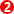﻿ Getting Started Tutorials - MechDesigner > Tutorial 14: Gear-Pairs > Fixed Gear and Orbiting Gear Arrangements > Option 1: Fixed Gear Centres > Reverted Gear-Train

# Reverted Gear-Train

### Reverted Gear-PairsSchematic of Reverted Gear-Train. A 'Reverted Gear Train' has: •two Gear-Pairs •two shafts •the input and output shafts are co-axialIt is a Compound Gear with, two Gears-Pairs, and a special condition that makes the total number of teeth of the first gear-pair equals the number of teeth of the second-gear-pair, when the module is the same. The image to the left shows a schematic of a Reverted Gear-Train. There is more than one gear-pair, two shafts, and the input and output shafts are co-axial. Therefore, it agrees the definition of the Reverted Gear Train.

#### Reverted Gear Train – Stage 1Start with a Gear-Pair with an External Mesh- see Option 1 Step 14.1 Here, the number-of-teeth are: T1=30, T2=70. The Gear Ratio is T1/T2 = 2.5 The total number-of-teeth is 100T.

#### Reverted Gear Train – Stage 21.Add a new Part 2.Add a Pin Joint: a.Click a Point in the new Part b.Click a Point on the Base-Part (left side of Line in Base-PartWe use the Point to add a Pin-Joint at the left end of the Line in the Base-Part two times - •»one time for the input gear, and •one time for the output gear.Add the second Gear-Pair. 1.Add Gear-Pair 2.Click the Part that is the Driven Geared-Rocker in the first Gear-Pair3.Click the new Parts with the Blue-Part-Outline4.The Line between the two Parts5.The dimension of the Line. This dimension is already a 'driven-dimension'.Edit the Gear-Pair 2 Edit the Number of Teeth: Gear 1 = 20 Gear 2 = 80 Video See Video to the left to see the gear reduction of the Gear-Pair. The Gear Reduction is : 30*20 : 70*80 = 3: 28